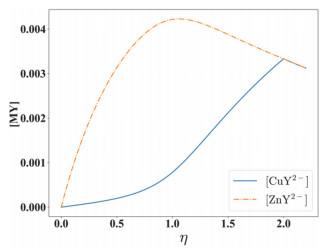## Some Explorations on the Introducing of Ideological and Political Elements into Analytical Chemistry Course

 基金资助: 中山大学2020年本科教学质量工程项目.  31000-18842502广东省研究生教育创新计划项目.  31000-18842212中山大学2020研究生培养综合改革专项.  31000-18842270国家自然科学基金.  22076222Abstract

Analytical chemistry has a breadth of audience in colleges and universities, because it is one of the basic courses for most disciplines. As analytical chemistry course starts at the early college year, it will play important influence on the formation of the correct ideological and political opinion of students. The authors made deep exploration on the ideological and political elements in classroom teaching of acid-base titration, complexation titration and Ringbom's equation. The results show that ideological and political elements can be integrated into teaching process if we hold the idea of cultural inheritance and innovation.

Keywords： Analytical chemistry ; Dialectical unity ; Quantitative change caused qualitative change ; Ideological and political elements ; Classroom teaching

Gan Feng. Some Explorations on the Introducing of Ideological and Political Elements into Analytical Chemistry Course. University Chemistry[J], 2021, 36(3): 2012009-0 doi:10.3866/PKU.DXHX202012009

### 1.1 从共轭酸碱定义的深度剖析提升学生的认知水平

1923年，布朗斯特(N. Brønsted)提出酸碱质子理论，定义酸是质子的供体，碱是质子的受体。酸给出质子后变成了它的共轭碱，碱接受质子后变成了它的共轭酸。这个定义的出现是为了解决阿伦尼乌斯(S. A. Arrhenius)提出的酸碱电离理论的不足而提出的一个新的酸碱定义。酸碱电离理论的框架中，把电离出的阴离子全部都是OH-的物质定义为碱。然而，越来越多的化学事实证明，很多的物质会导致其水溶液中OH-的增加，但是这种增加不是该物质电离出OH-，而是因为它们夺取了水中的氢离子，由此导致水溶液中的OH-的增加。

$K_{\mathrm{a}}(\mathrm{HB}) \times K_{\mathrm{b}}\left(\mathrm{B}^{-}\right)=1.0 \times 10^{-14}$

$\delta\left(\mathrm{NH}_{3}\right)=\frac{K_{\mathrm{a}}\left(\mathrm{NH}_{4}^{+}\right) c^{\ominus}}{\left[\mathrm{H}^{+}\right]+K_{\mathrm{a}}\left(\mathrm{NH}_{4}^{+}\right) c^{\ominus}}$

$\delta\left(\mathrm{NH}_{4}^{+}\right)=\frac{\left[\mathrm{H}^{+}\right]}{\left[\mathrm{H}^{+}\right]+K_{\mathrm{a}}\left(\mathrm{NH}_{4}^{+}\right) c^{\ominus}}$

### 1.2 从酸碱滴定通式的建立过程领会从量变到质变

$\eta=-\frac{\sum F_{\mathrm{s}} c_{\mathrm{s}}+\Delta}{\sum F_{\mathrm{t}} c_{\mathrm{t}}+\Delta}$

$\eta=\frac{-F(\mathrm{HCl}) c(\mathrm{HCl})-\Delta}{c(\mathrm{NaOH})+\Delta}=-\frac{F(\mathrm{HCl}) c(\mathrm{HCl})+\Delta}{c(\mathrm{NaOH})+\Delta}$

$\eta=\frac{-F(\mathrm{HAc}) c(\mathrm{HAc})-\Delta}{c(\mathrm{NaOH})+\Delta}=-\frac{F(\mathrm{HAc}) c(\mathrm{HAc})+\Delta}{c(\mathrm{NaOH})+\Delta}$

$\eta=\frac{-F\left(\mathrm{H}_{3} \mathrm{PO}_{4}\right) c\left(\mathrm{H}_{3} \mathrm{PO}_{4}\right)-\Delta}{c(\mathrm{NaOH})+\Delta}=-\frac{F\left(\mathrm{H}_{3} \mathrm{PO}_{4}\right) c\left(\mathrm{H}_{3} \mathrm{PO}_{4}\right)+\Delta}{c(\mathrm{NaOH})+\Delta}$

$\eta=-\frac{F(\mathrm{HCl}) c(\mathrm{HCl})+F(\mathrm{HAc}) c(\mathrm{HAc})+F\left(\mathrm{H}_{3} \mathrm{PO}_{4}\right) c\left(\mathrm{H}_{3} \mathrm{PO}_{4}\right)+\Delta}{c(\mathrm{NaOH})+\Delta}$

### 图1### 2.2 以配位滴定通式的建立过程激发学生的“创新意识”和“文化自信”

$\eta=\frac{\alpha(\mathrm{Y} \cdot \mathrm{H})+\sum_{i=1}^{n} \frac{K\left(\mathrm{M}_{i} \mathrm{Y}\right)[\mathrm{Y}] c\left(\mathrm{M}_{i}\right)}{\alpha\left(\mathrm{M}_{i}\right) c^{\ominus}+K\left(\mathrm{M}_{i}\right)[\mathrm{Y}]}}{c(\mathrm{Y})-\alpha(\mathrm{Y} \cdot \mathrm{H})[\mathrm{Y}]}$

$\left[\mathrm{M}_{i}\right]=\frac{c\left(\mathrm{M}_{i}\right)}{(1+\eta)\left(\alpha\left(\mathrm{M}_{i}\right)+K\left(\mathrm{M}_{i} \mathrm{Y}\right) \frac{[\mathrm{Y}]}{c^{\ominus}}\right)}, i=1, 2, \ldots, n.$

### 图2### 图3## 3 滴定终点误差计算式中的课程思政元素

2019年，本文作者发表了一篇论文，对林帮公式再度进行讨论。本文作者的做法是直接以终点误差的主流定义为基准，从数学上揭示林帮公式的本质。滴定终点误差的主流定义如下：

$(\mathrm{TE} \%)_{\text {主流 }}=\frac{\text { 终点时滴定剂过量 }(\text { 或不足 }) \text { 的摩尔数 }}{\text { 待测物质的摩尔数 }}=\frac{c(\mathrm{~L}) V_{\mathrm{ep}}-c(\mathrm{M}) V_{0}}{c(\mathrm{M}) V_{0}}$

\begin{aligned}(\mathrm{TE} \%)_{\text {林帮 }} &=\frac{[\mathrm{L}]_{\mathrm{ep}}-[\mathrm{M}]_{\mathrm{ep}}}{C_{\mathrm{M}}} \\ &=\frac{\frac{c(\mathrm{~L}) V_{\mathrm{ep}}-c(\mathrm{M}) V_{0}}{V_{\mathrm{ep}}+V_{0}}}{\frac{c(\mathrm{M}) V_{0}}{V_{\mathrm{sp}}+V_{0}}} \\ &=\frac{V_{\mathrm{sp}}+V_{0}}{V_{\mathrm{ep}}+V_{0}} \times \frac{c(\mathrm{~L}) V_{\mathrm{ep}}-c(\mathrm{M}) V_{0}}{c(\mathrm{M}) V_{0}} \\ &=\frac{V_{\mathrm{sp}}+V_{0}}{V_{\mathrm{ep}}+V_{0}} \times(\mathrm{TE} \%)_{\text {主流 }} \end{aligned}

## 参考文献 原文顺序 文献年度倒序 文中引用次数倒序 被引期刊影响因子

De Levie R. Anal. Chem. 1996, 68, 585.

Gan F. ; Chu Y. X. ; Yang W. L. Chemom. Intell. Lab. Systs. 2020, 203, 104069.

Ringbom, A. 分析化学中的络合作用. 戴明, 译. 北京: 高等教育出版社, 1987.

/

 〈〉